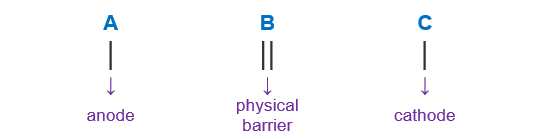# Problem: Use line notation to represent each electrochemical cellO2(g) + 4 H+ (aq) + 2 Zn(s)→ 2 H2O(l) + 2Zn2+(aq)

###### FREE Expert Solution

We’re being asked to determine the cell notation for the redox reaction shown below:

O2(g) + 4 H(aq) + 2 Zn(s)→ 2 H2O(l) + 2Zn2+(aq)

When writing a cell notation, we use the following format – “as easy as ABCTo determine the cell notation of the reaction, we will use the following steps:

Step 1: Separate the reaction into two half-reactions.
Step 2: Determine the anode and the cathode.
Step 3: Write the cell notation of the reaction.

83% (45 ratings)###### Problem Details

Use line notation to represent each electrochemical cell

O2(g) + 4 H+ (aq) + 2 Zn(s)→ 2 H2O(l) + 2Zn2+(aq)